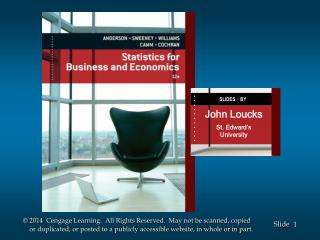DownloadDownload PresentationJohn Loucks St . Edward’s University

# John Loucks St . Edward’s University

Télécharger la présentation## John Loucks St . Edward’s University

- - - - - - - - - - - - - - - - - - - - - - - - - - - E N D - - - - - - - - - - - - - - - - - - - - - - - - - - -
##### Presentation Transcript

1. SLIDES.BY . . . . . . . . . . . John Loucks St. Edward’s University

2. Chapter 14, Part B Simple Linear Regression • Using the Estimated Regression Equation for Estimation and Prediction • Computer Solution • Residual Analysis: Validating Model Assumptions • Residual Analysis: Outliers and Influential • Observations

3. Using the Estimated Regression Equationfor Estimation and Prediction • A confidence interval is an interval estimate of the • mean value of y for a given value of x. • A prediction interval is used whenever we want to • predict an individual value of y for a new observation • corresponding to a given value of x. • The margin of error is larger for a prediction interval.

4. Using the Estimated Regression Equationfor Estimation and Prediction • Confidence Interval Estimate of E(y*) • Prediction Interval Estimate of y* where: confidence coefficient is 1 -  and t/2 is based on a t distribution with n - 2 degrees of freedom

5. ^ y = 10 + 5(3) = 25 cars Point Estimation If 3 TV ads are run prior to a sale, we expect the mean number of cars sold to be:

6. Confidence Interval for E(y*) • Estimate of the Standard Deviation of

7. Confidence Interval for E(y*) The 95% confidence interval estimate of the mean number of cars sold when 3 TV ads are run is: 25 + 3.1824(1.4491) 25 + 4.61 20.39 to 29.61 cars

8. Prediction Interval for y* • Estimate of the Standard Deviation of an Individual Value of y*

9. Prediction Interval for y* The 95% prediction interval estimate of the number of cars sold in one particular week when 3 TV ads are run is: 25 + 3.1824(2.6013) 25 + 8.28 16.72 to 33.28 cars

10. Computer Solution • Performing the regression analysis computations • without the help of a computer can be quite time • consuming. • On the next slide we show Minitab output for the • Reed Auto Sales example. • Recall that the independent variable was named Ads • and the dependent variable was named Cars in the • example.

11. Computer Solution Estimated Regression Equation • Minitab • Output ANOVA Table Interval Estimates

12. Minitab Output • Minitab prints the estimated regression equation as • Cars = 10.0 + 5.00 Ads. • For each of the coefficients b0 and b1, the output shows • its value, standard deviation, t value, and p-value. • Minitab prints the standard error of the estimate, s, • as well as information about the goodness of fit. . • The standard ANOVA table is printed. • Also provided are the 95% confidence interval • estimate of the expected number of cars sold and the • 95% prediction interval estimate of the number of • cars sold for an individual weekend with 3 ads.

13. Residual Analysis • If the assumptions about the error term e appear • questionable, the hypothesis tests about the • significance of the regression relationship and the • interval estimation results may not be valid. • The residuals provide the best information about e. • Residual for Observation i • Much of the residual analysis is based on an • examination of graphical plots.

14. Residual Plot Against x • If the assumption that the variance of e is the same for all values of x is valid, and the assumed regression model is an adequate representation of the relationship between the variables, then • The residual plot should give an overall • impression of a horizontal band of points

15. Residual Plot Against x Good Pattern Residual 0 x

16. Residual Plot Against x Nonconstant Variance Residual 0 x

17. Residual Plot Against x Model Form Not Adequate Residual 0 x

18. Residual Plot Against x • Residuals

19. Residual Plot Against x

20. Standardized Residuals • Standardized Residual for Observation i where:

21. Standardized Residual Plot • The standardized residual plot can provide insight about the assumption that the error term e has a normal distribution. • If this assumption is satisfied, the distribution of the standardized residuals should appear to come from a standard normal probability distribution.

22. Standardized Residual Plot • Standardized Residuals

23. Standardized Residual Plot • Standardized Residual Plot

24. Standardized Residual Plot • All of the standardized residuals are between –1.5 and +1.5 indicating that there is no reason to question the assumption that e has a normal distribution.

25. Outliers and Influential Observations • Detecting Outliers • An outlier is an observation that is unusual in comparison with the other data. • Minitab classifies an observation as an outlier if its standardized residual value is < -2 or > +2. • This standardized residual rule sometimes fails to identify an unusually large observation as being an outlier. • This rule’s shortcoming can be circumvented by using studentized deleted residuals. • The |i th studentized deleted residual| will be larger than the |i th standardized residual|.

26. End of Chapter 14, Part B